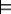### 6.1.1 Semantics of Probability

Probability theory is built on the same foundation of worlds and variables as constraint satisfaction (see Section 4.2). Instead of having constraints that eliminate some worlds and treat every other world as possible, probabilities put a measure over the possible worlds. The variables in probability theory are referred to as random variables. The term random variable is somewhat of a misnomer because it is neither random nor variable. As discussed in Section 4.2, worlds can be described in terms of variables; a world corresponds to an assignment of a value to each variable. Alternatively, variables can be described in terms of worlds; a variable is a function that returns a value on each world.

First we define probability as a measure on sets of worlds, then define probabilities on propositions, then on variables.

A probability measure over the worlds is a function µ from sets of worlds into the non-negative real numbers such that

• if Ω1 and Ω2 are disjoint sets of worlds (i.e., if Ω1∩Ω2={}), then µ(Ω1∪Ω2)=µ(Ω1)+µ(Ω2);
• if Ω is the set of all worlds, µ(Ω)=1.

Note that the use of 1 as the probability of the set of all of the worlds is just by convention. You could just as well use 100. It is possible to have infinitely many worlds when some variables have infinite domains or when infinitely many variables exist. When there are infinitely many worlds, we do not require a measure for all subsets of Ω - just for those sets that can be described using some language that we assume allows us to describe the intersection, union, and complement of sets. The set of subsets describable by these operations has the structure of what mathematicians call an algebra.

The measure µ can be extended to worlds by defining µ(ω)=µ({ω}) for world ω. When finitely many worlds exist, the measure over individual worlds is adequate to define µ. When infinitely many worlds exist, it is possible that the measure of individual worlds is not enough information to define µ, or that it may not make sense to have a measure over individual worlds.

Example 6.2: Suppose the worlds correspond to the possible real-valued heights, in centimeters, of a particular person. In this example, there are infinitely many possible worlds. The measure of the set of heights in the range [175,180) could be 0.2 and the measure of the range [180,190) could be 0.3. Then the measure of the range [175,190) is 0.5. However, the measure of any particular height could be zero.

As described in Section 5.1, a primitive proposition is an assignment of a value to a variable. Propositions are built from primitive propositions using logical connectives. We then use this property to define probability distributions over variables.

The probability of proposition α, written P(α), is the measure of the set of possible worlds in which α is true. That is,

P(α)=µ({ω: ωα} ),

where ωα means α is true in world ω. Thus, P(α) is the measure of the set of worlds in which α is true.

This use of the symboldiffers from its use in the previous chapter (see Section 5.1). There, the left-hand side was a knowledge base; here, the left-hand side is a world. Which meaning is intended should be clear from the context.

A probability distribution, P(X), over a random variable X is a function from the domain of X into the real numbers such that, given a value x∈dom(X), P(x) is the probability of the proposition X=x. We can also define a probability distribution over a set of variables analogously. For example, P(X,Y) is a probability distribution over X and Y such that P(X=x,Y=y), where x∈dom(X) and y ∈dom(Y), has the value P(X=x∧Y=y), where X=x∧Y=y is a proposition and P is the function on propositions. We will use probability distributions when we want to treat a set of probabilities as a unit.

Probability Density Functions

The definition of probability is sometimes specified in terms of probability density functions when the domain is continuous (e.g., a subset of the real numbers). A probability density function provides a way to give a measure over sets of possible worlds. The measure is defined in terms of an integral of a probability density function. The formal definition of an integral is the limit of the discretizations as the discretizations become finer.

The only non-discrete probability distribution we will use in this book is where the domain is the real line. In this case, there is a possible world for each real number. A probability density function, which we write as p, is a function from reals into non-negative reals that integrates to 1. The probability that a real-valued random variable X has value between a and b is given by

P(a ≤ X ≤ b)=∫ab p(X ) dX .

A parametric distribution is one where the density function can be described by a formula. Although not all distributions can be described by formulas, all of the ones that we can represent are. Sometimes statisticians use the term parametric to mean the distribution can be described using a fixed, finite number of parameters. A non-parametric distribution is one where the number of parameters is not fixed. (Oddly, non-parametric typically means "many parameters").

A common parametric distribution is the normal or Gaussian distribution with mean µ and variance σ2 defined by where σ is the standard deviation. The normal distribution is used for measurement errors, where there is an average value, given by µ, and a variation in values specified by σ. The central limit theorem, proved by Laplace(1812), specifies that a sum of independent errors will approach the Gaussian distribution. This and its nice mathematical properties account for the widespread use of the normal distribution.

Other distributions, including the beta and Dirichlet distributions, are discussed in the Learning Under Uncertainty section.